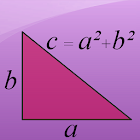Pythagorean Theorem Calculator

• SEARCH TYPE

All Android applications categories

All Android games categories# Pythagorean Theorem Calculator

by: 1.2K 8

8 Users
rating

## Screenshots

Description

This free math calculator will instantly solve the Pythagorean equation. The Pythagorean Theorem states that a²+b²=c² and can be used to find the length of the hypotenuse of a right triangle. Simply input the length of two sides and the app will calculate the third side according to the Pythagorean Theorem.
For example enter side A and sides B and the calculator will calculate the length of side C.

The best app for school and college! If you are a student, it will help you to learn algebra and geometry!

Tags: 勾股定理 , baixar gratis descrobindo o teorema de pitagoras , bwulr , pitagoras math , teorema di pitagora con calculator

## Users review

from 1.235 reviews

"Great"

8